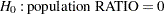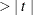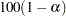### Ratio Analysis

The "Ratio Analysis" table displays statistics for all the ratios that you request in the RATIO statement. If you do not specify any statistic-keywords in the PROC SURVEYMEANS  statement, then by default this table displays the ratios and standard errors. The "Ratio Analysis" table can contain the following information for each ratio, depending on which statistic-keywords you request:

• Numerator, which identifies the numerator variable of the ratio

• Denominator, which identifies the denominator variable of the ratio

• N, which is the number of observations used in the ratio analysis

• number of Clusters

• Sum of Weights

• DF, which is the degrees of freedom for thetest

• Ratio

• Std Err of Ratio, which is the standard error of the ratio

• Var, which is the variance of the ratio

•Value, for testing• Pr, which is the two-sided-value for thetest

•% CL for Ratio, which are two-sided confidence limits for the Ratio

• Upper% CL for Ratio, which are one-sided upper confidence limits for the Ratio

• Lower% CL for Ratio, which are one-sided lower confidence limits for the Ratio

When you use the ODS OUTPUT statement to create an output data set, if you use labels for your RATIO statement, these labels are saved in the variable Ratio Statement in the output data set.